﻿• 价格透明
• 信息保密
• 进度掌控
• 售后无忧• 学习目标：
• 学习内容：
• 题目描述
• 解题思路：
• 实现代码

# 题目描述

max]上，如果有数不可以被arr某一个子集相加得到，那么其中最小的那个数是arr的最小不可组成和 在区间[min,
max]上，如果所有的数都可以被arr的某一个子集相加得到，那么max+1是arr的最小不可组成和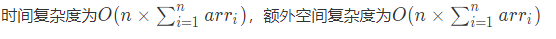3
2 3 9

4

3
1 2 4

8

3 = 1 + 2
5 = 1 + 4
6 = 2 + 4
7 = 1 + 2 + 4

# 解题思路：

ary[]表示给定的数组
F(i,j):表示ary的前 i+1 个数字是否有和为 j 的组成序列

F(i,0)=true 表示和是零一定能得到
F(0,ary)=true ：表示可以得到以第一个元素为和的序列（只包含第一个元素）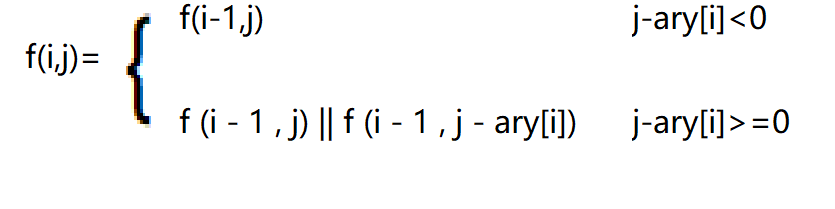j - ary[ i ] < 0表示：当前和 j 小于当前位置元素 ary[i] ,不能通过包含当前位置元素的序列得到
j - ary[ i ] >= 0表示：当前和 j 大于等于当前位置元素 ary[i] ,可能可以通过包含当前位置元素的序列得到

1. 初始化二维数组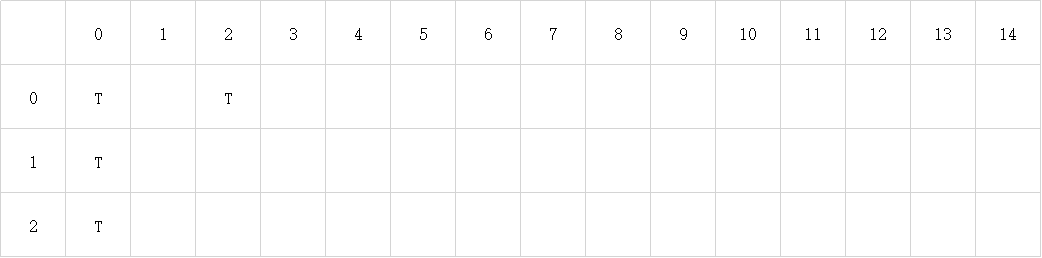表示前i+1个数字 { 2 } 可以得到的和是 0 ，2两个值
2. 第一步循环，得到第二行的可组成和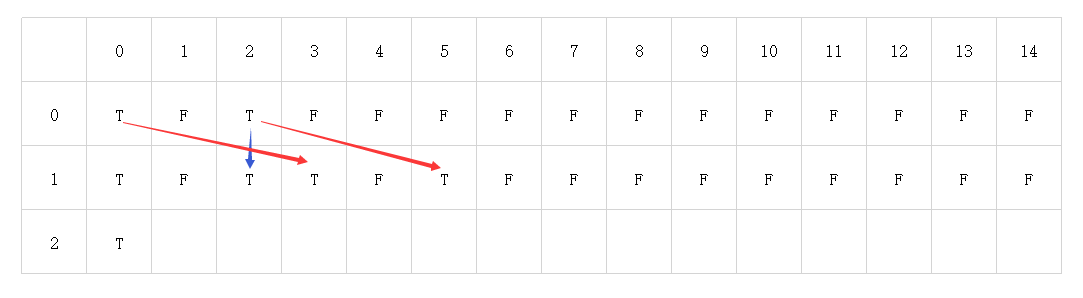1. 第二步循环，得到第三行的值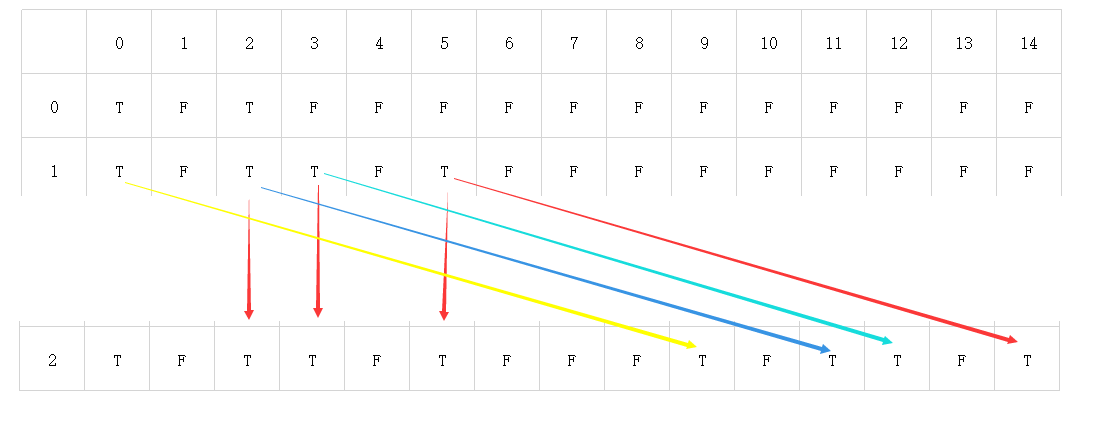（由于线太多，所以我把图片分开了 (｀・ω・´) ）

# 实现代码

1. 二维数组实现：
``````import java.util.Scanner;

public class Main {
public static void main(String[] args) {
Scanner sc = new Scanner(System.in);
while (sc.hasNext()) {
int n = sc.nextInt();
int[] ary = new int[n];
for (int i = 0; i < n; i++) {
ary[i] = sc.nextInt();
}
System.out.println(getFirstUnFormedNum(ary));
}
}

public static int getFirstUnFormedNum(int[] ary) {
int min = Integer.MAX_VALUE;//记录最小和
int max = 0;//记录最大和
for (int i = 0; i < ary.length; i++) {
max += ary[i];
min = Math.min(min, ary[i]);
}
boolean[][] res = new boolean[ary.length][max + 1];
for (int i = 0; i < ary.length; i++) {
//初始化第一列
res[i] = true;
}
res[ary] = true;//初始化第一行，其他位置默认是false
for (int i = 1; i < res.length; i++) {
for (int j = 1; j < res.length; j++) {
if (j - ary[i] < 0) {
//F(i,j)=F(i-1,j)
res[i][j] = res[i - 1][j];
} else {
//F(i,j)=F(i-1,j)||F(i-1,j-ary[i])
res[i][j] = res[i - 1][j] || res[i - 1][j - ary[i]];
}
}
}
for (int i = min; i < res.length; i++) {
if (!res[res.length - 1][i]) {
return i;
}
}
return res.length;
}
}
``````
1. 代码优化，一位数组实现
``````import java.util.Scanner;

public class Main {
public static void main(String[] args) {
Scanner sc = new Scanner(System.in);
while (sc.hasNext()) {
int n = sc.nextInt();
int[] ary = new int[n];
for (int i = 0; i < n; i++) {
ary[i] = sc.nextInt();
}
System.out.println(getFirstUnFormedNum(ary));
}
}
public static int getFirstUnFormedNum(int[] arr) {
int min = Integer.MAX_VALUE;
int max = 0;
for (int i = 0; i < arr.length; i++) {
max += arr[i];
min = Math.min(min, arr[i]);
}
boolean res[] = new boolean[max + 1];
res = true; // 为了使单个元素去求和时是真的 (i + 0 = i)
for (int i = 0; i < arr.length; i++) {
for (int j = max; j >= arr[i]; j--) {
res[j] = res[j - arr[i]] || res[j];
}
}
for (int i = min; i < res.length; i++) {
if (!res[i])
return i;
}
return max + 1;
}
}

``````### 低价透明### 金牌服务### 信息保密